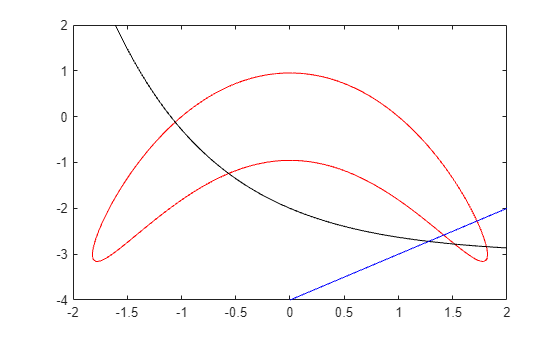# Solve Nonlinear Feasibility Problem, Problem-Based

This example shows how to find a point that satisfies all the constraints in a problem, with no objective function to minimize.

### Problem Definition

For example, suppose that you have the following constraints:

`$\begin{array}{l}\left(y+{x}^{2}{\right)}^{2}+0.1{y}^{2}\le 1\\ y\le \mathrm{exp}\left(-x\right)-3\\ y\le x-4.\end{array}$`

Do any points $\left(x,y\right)$ satisfy all of the constraints?

### Problem-Based Solution

Create an optimization problem that has only constraints, no objective function.

```x = optimvar('x'); y = optimvar('y'); prob = optimproblem; cons1 = (y + x^2)^2 + 0.1*y^2 <= 1; cons2 = y <= exp(-x) - 3; cons3 = y <= x - 4; prob.Constraints.cons1 = cons1; prob.Constraints.cons2 = cons2; prob.Constraints.cons3 = cons3; show(prob)```
``` OptimizationProblem : Solve for: x, y minimize : subject to cons1: ((y + x.^2).^2 + (0.1 .* y.^2)) <= 1 subject to cons2: y <= (exp(-x) - 3) subject to cons3: y - x <= -4 ```

Create a pseudorandom start point structure `x0` with fields `x` and `y` for the optimization variables.

```rng default x0.x = randn; x0.y = randn;```

Solve the problem starting from `x0`.

`[sol,~,exitflag,output] = solve(prob,x0)`
```Solving problem using fmincon. Local minimum found that satisfies the constraints. Optimization completed because the objective function is non-decreasing in feasible directions, to within the value of the optimality tolerance, and constraints are satisfied to within the value of the constraint tolerance. ```
```sol = struct with fields: x: 1.7903 y: -3.0102 ```
```exitflag = OptimalSolution ```
```output = struct with fields: iterations: 6 funcCount: 9 constrviolation: 0 stepsize: 0.2906 algorithm: 'interior-point' firstorderopt: 0 cgiterations: 0 message: '...' bestfeasible: [1x1 struct] objectivederivative: "closed-form" constraintderivative: "forward-AD" solver: 'fmincon' ```

The solver finds a feasible point.

### Initial Point Importance

The solver can fail to find a solution when starting from some initial points. Set the initial point `x0.x = -1`, `x0.y = -4` and solve the problem starting from `x0`.

```x0.x = -1; x0.y = -4; [sol2,~,exitflag2,output2] = solve(prob,x0)```
```Solving problem using fmincon. Converged to an infeasible point. fmincon stopped because it is unable to find a point locally that satisfies the constraints within the value of the constraint tolerance. ```
```sol2 = struct with fields: x: -2.1266 y: -4.6657 ```
```exitflag2 = NoFeasiblePointFound ```
```output2 = struct with fields: iterations: 121 funcCount: 279 constrviolation: 1.4609 stepsize: 1.9997e-10 algorithm: 'interior-point' firstorderopt: 0 cgiterations: 261 message: '...' bestfeasible: [] objectivederivative: "closed-form" constraintderivative: "forward-AD" solver: 'fmincon' ```

Check the infeasibilities at the returned point.

`inf1 = infeasibility(cons1,sol2)`
```inf1 = 1.1974 ```
`inf2 = infeasibility(cons2,sol2)`
```inf2 = 0 ```
`inf3 = infeasibility(cons3,sol2)`
```inf3 = 1.4609 ```

Both `cons1` and `cons3` are infeasible at the solution `sol2`. The results highlight the importance of using multiple start points to investigate and solve a feasibility problem.

### Visualize Constraints

To visualize the constraints, plot the points where each constraint function is zero by using `fimplicit`. The `fimplicit` function passes numeric values to its functions, whereas the `evaluate` function requires a structure. To tie these functions together, use the `evaluateExpr` helper function, which appears at the end of this example. This function simply puts passed values into a structure with the appropriate names.

Note: If you use the live script file for this example, the `evaluateExpr` function is already included at the end of the file. Otherwise, you need to create this function at the end of your .m file or add it as a file on the MATLAB® path.

Avoid a warning that occurs because the `evaluateExpr` function does not work on vectorized inputs.

```s = warning('off','MATLAB:fplot:NotVectorized'); cc1 = (y + x^2)^2 + 0.1*y^2 - 1; fimplicit(@(a,b)evaluateExpr(cc1,a,b),[-2 2 -4 2],'r') hold on cc2 = y - exp(-x) + 3; fimplicit(@(a,b)evaluateExpr(cc2,a,b),[-2 2 -4 2],'k') cc3 = y - x + 4; fimplicit(@(x,y)evaluateExpr(cc3,x,y),[-2 2 -4 2],'b') hold off````warning(s);`

The feasible region is inside the red outline and below the black and blue lines. The feasible region is at the lower right of the red outline.

### Helper Function

This code creates the `evaluateExpr` helper function.

```function p = evaluateExpr(expr,x,y) pt.x = x; pt.y = y; p = evaluate(expr,pt); end```